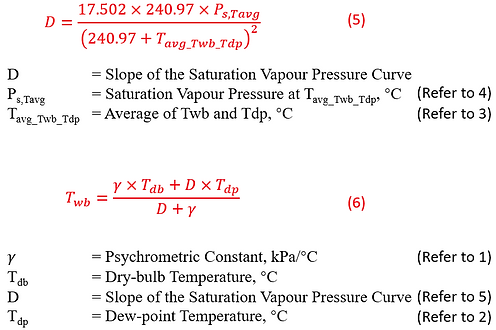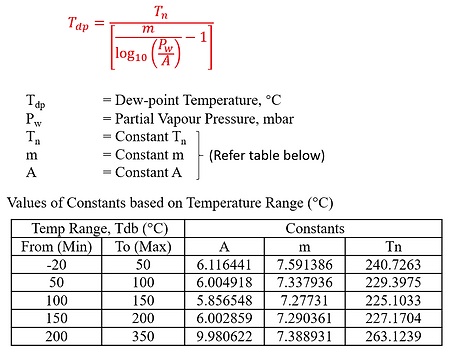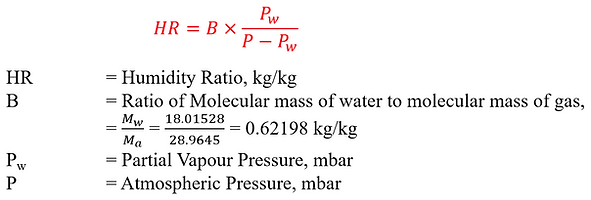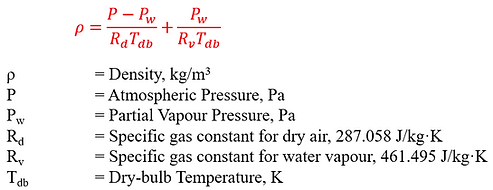This calculator is an enhancement for Psychrometric Calculator which mathematically approximates values from the psychrometric chart with acceptable degree of accuracy.

There can be many different ways to compute different parameters of air properties, each to a certain degree of difficulties. The purpose of this page is to explain the formulae (and the ways) these parameters can be represented (with acceptable degree of accuracy) in formulae. To prove that these formulae work, an Excel file is attached with each of the parameters of air properties we will see in a psychrometric calculator.

In order to use the Calculator, unzip the downloaded document, and make sure to enable macros in your Excel. Note that the Excel built-in Solver function is used by the calculator, which may take some time to produce an answer. Make sure to enable solver function and enable iteration in "Options" (see detailed explanation here).

From the Excel File, the calculator consists of 2 parts

1. Part 1 computes with dry-bulb temperature, Tdb, and relative humidity, RH as the input parameters

2. Part 2 computes with dry-bulb temperature, Tdb, and wet-bulb temperature, Twb as the input parameters

In the following section, we will disclose the formulae involved for the computation of all parameters of air properties in this psychrometric calculator. Important air properties include:

1. Dry-bulb temperature, Tdb

2. Wet-bulb temperature, Twb

3. Relative Humidity, RH

4. Atmospheric Pressure, P

5. Saturation Vapour Pressure, Pws

6. Partial Vapour Pressure, Pw

7. Dew-point temperature, Tdp

8. Humidity Ratio, HR

9. Enthalpy, h

10. Density, ρ

11. Specific volume of moist air per mass of dry air, Vda

12. Specific volume of air, V

Apart from the theory and formulae, if you are interested to learn about the programming behind this excel calculator, you may visit this page: Using Excel Macros to compute Air Properties.

Part 1: Calculating Air Properties from dry-bulb temperature, Tdb and relative humidity, RH

Wet-bulb temperature, Twb

To calculate Twb from Tdb and RH, there are 3 popular methods as shown below.

Method 1: Using the ATAN formulaTWB =TDB*ATAN(0.151977*(RH+8.313659)^0.5)+ATAN(TDB+RH)-ATAN(RH-1.676331)+0.00391838*RH^1.5*ATAN(0.023101*RH)-4.686035

Where

TWB = Wet-bulb Temperature (°C)

TDB = Dry-bulb Temperature (°C)

RH = Relative Humidity (%)

Method 2: Using Excel Solver for the Model developed by Al-Ismaili and Al-Azri (2016)

While this method is elaborated in detail in the link below, the following section will describe briefly how the calculation is worked.

AL-ISMAILI: Simple iterative approach to calculate... - Google Scholar

The theory is simple. We want to calculate Twb from Tdb and RH, by equating the humidity ratio (HR) from Equation (1) and (2). In order for both HR’s to be equal, we reiterate the value of Twb (variable in Excel Solver) until the difference between the 2 HR approximates to 0 (objective in Excel Solver). This can be done using the Excel Solver function, which can be triggered with a button assigned to a Macro (VBA).Method 3: Using Excel Circular References

This method of computation requires iterative calculations to be enabled. (File > Options > Formula > Enable Iterative Calculation)This method of calculation is explained by Jay Ham from the link address below, and the formulae involved are as follow:

Calculating Wet Bulb Temperature From Weather Data Using Excel - YouTubeAtmospheric Pressure, P

The atmospheric pressure is expressed in terms of the elevation (altitude) above sea level, sea level temperature and pressure. Nonetheless, the International Standard Atmosphere (ISA) model defines standard sea level pressure at 101.325 kPa and 59°F (15°C) without reference to season or regions. As the atmospheric pressure decreases with altitude, the temperature decreases at a standard lapse rate. The computation for atmospheric pressure based on altitude is further elaborated here.

Meanwhile, a simpler, less comprehensive but reliable calculation method can be performed through the formula shown below:Saturation Vapour Pressure, Pws

The Saturation Vapour Pressure, Pws can be computed with acceptable accuracy between 0°C to 373°C, with reference to (W. Wagner and A. Pruß:" The IAPWS Formulation 1995 for the Thermodynamic Properties of Ordinary Water Substance for General and Scientific Use")

The computation is rather straightforward, with the steps elaborated clearly below:Alternatively, the method proposed by Al-Ismaili and Al-Azri (2016) for the computation of Twb shown above (Copied below) may also provide a good approximation.Partial Vapour Pressure, Pw

The partial vapour pressure, Pw can be easily derived from Relative Humidity, RH, and the water vapour saturation pressure, PwsDew-point temperature, Tdp

With the partial vapour pressure, Pw computed from the previous step, the dew-point temperature, Tdp can be estimated using the formula below. The values of constants Tn, m and A shall be referred to the Table below based on the ambient temperature range. For example, if the dry-bulb temperature, Tdb is 25°C, we shall select values from the first row, i.e. A=6.116441, m=7.591386, Tn=240.7263.Alternatively, the method shown in the computation of Twb shown above (copied below) may also be applicable.

Humidity Ratio, HR

Humidity Ratio, HR (or mixing ratio) can be computed with the formula shown below, expressed in kg/kg. To express HR in g/kg, simply multiply B by 1000.This is similar to the formula used in the computation of Twb shown in the section above (copied below).

Enthalpy, h

Enthalpy, h can be derived from dry-bulb temperature, Tdb and Humidity Ratio, HR (note: in g/kg) represented in the formula as shown below.You may wonder why there are discrepancies when we convert Enthalpy from Imperial (btu/lb) to S.I. units (kJ/kg) or vice-versa. This is explained by the fact that there is no absolute scale for Enthalpy. Zero Enthalpy on the Imperial Chart is referenced at 0°F, whereas that on the Metric Chart is based on 0°C. Since different calculations may assume a different reference point, it is never valid to measure Enthalpy under 1 condition, i.e., it is only valid to compute a difference between 2 measured Enthalpy.

Density, ρ

The density of the air, ρ can be calculated from the equation below. The following formula is derived from Omnicalculator website at https://www.omnicalculator.com/physics/air-densitySpecific volume of moist air per mass of dry air, vda

The specific volume of moist air per mass of dry air, vda is often confused with specific volume of air, v.

It is worth taking note that HVAC calculations with variable specific volume were all derived based on the definition of specific volume referenced to a unit mass of dry air. Therefore, specific volume of moist air per unit mass of dry air, vda (instead of v) shall be the desired property in most HVAC applications.

The following equation may be useful to compute vda, while relating vda to v. From the equation, we know that the specific volume of moist air per mass of dry air, vda, is not the reciprocal of density, ρ. Specific volume, v is. More to read: https://daytonashrae.org/psychrometrics/psychrometrics_sv.html

Part 2: Calculating Air Properties from dry-bulb temperature, Tdb and wet-bulb temperature, Twb

Relative Humidity, RH

Method 1: Using the straightforward approximation methodMethod 2: Using Pw and Pws

RH can be derived from equation (4) above, where RH = Pw/Pws x 100%.

Pw and Pws can be calculated from Method 1 just above, or from the method shown in the following sections below –

Method 3: Using Excel Solver for the Model developed by Al-Ismaili and Al-Azri (2016)

The exact same method for the computation of Twb from Tdb and RH (part 1) can be applied for the computation of RH from Tdb and Twb.

As a recap, in part 1, we want to calculate Twb from Tdb and RH, by equating the humidity ratio (HR) from Equation (1) and (2) shown below. In order for both HR’s to be equal, we reiterate the value of Twb until the difference between the 2 HR's approximates to 0.

Now, in part 2, we want to calculate RH from Tdb and Twb by equating the humidity ratio (HR) from the same equations (1) and (2). However, in this case, in order for both HR’s to be equal, we reiterate the value of Relative Humidity (%), RH until the difference between the 2 HR's approximates to 0.Atmospheric Pressure, P

The computation of atmospheric pressure from elevation (altitude) above sea level, sea level temperature and pressure can be referred to the above equation in Part 1.

Saturation Vapour Pressure, Pws

The computation of saturation vapour pressure can be referred to the above equation in Part 1.

Partial Vapour Pressure, Pw

Apart from that, equation (3) from the computation of RH, Method 1 in Part 2 above (copied below), describes method (2) and method (3) as 2 methods that gives good approximation for partial vapour pressure.Method 2

Partial Vapour Pressure can be computed from the equation as shown below.The saturation vapour pressure at wet-bulb temperature, Pws(Twb) can be computed from the equation above in Part 1, but substituting Tdb with Twb as shown below.Method 3

Refer to Using Excel Solver for the Model developed by Al-Ismaili and Al-Azri (2016) (Part 1 Method 2) for the computation of Pw.

Dew-point temperature, Tdp

The computation of dew-point temperature, Tdp can be referred to the above equation in Part 1. The value of Pw used in the computation can either be derived from method 2 or method 3 elaborated above.

Humidity Ratio, HR

The computation of humidity ratio, HR can be referred to the above equation in Part 1. The value of Pw used in the computation can either be derived from method 2 or method 3 elaborated above.

Enthalpy, h

The computation of Enthalpy, h can be referred to the above equation in Part 1. The value of Pw used in the computation can either be derived from method 2 or method 3 elaborated above.

Density, ρ

The computation of Density, ρ can be referred to the above equation in Part 1. The value of Pw used in the computation can either be derived from method 2 or method 3 elaborated above.

Specific volume of moist air per mass of dry air, vda

The computation of Specific volume of moist air per mass of dry air, vda can be referred to the above equation in Part 1. The value of Pw used in the computation can either be derived from method 2 or method 3 elaborated above.